/scikit-learn

# sklearn.metrics.classification_report

`sklearn.metrics.classification_report(y_true, y_pred, labels=None, target_names=None, sample_weight=None, digits=2, output_dict=False)` [source]

Build a text report showing the main classification metrics

Read more in the User Guide.

Parameters: `y_true : 1d array-like, or label indicator array / sparse matrix` Ground truth (correct) target values. `y_pred : 1d array-like, or label indicator array / sparse matrix` Estimated targets as returned by a classifier. `labels : array, shape = [n_labels]` Optional list of label indices to include in the report. `target_names : list of strings` Optional display names matching the labels (same order). `sample_weight : array-like of shape = [n_samples], optional` Sample weights. `digits : int` Number of digits for formatting output floating point values. When `output_dict` is `True`, this will be ignored and the returned values will not be rounded. `output_dict : bool (default = False)` If True, return output as dict `report : string / dict` Text summary of the precision, recall, F1 score for each class. Dictionary returned if output_dict is True. Dictionary has the following structure: ```{'label 1': {'precision':0.5, 'recall':1.0, 'f1-score':0.67, 'support':1}, 'label 2': { ... }, ... } ``` The reported averages include micro average (averaging the total true positives, false negatives and false positives), macro average (averaging the unweighted mean per label), weighted average (averaging the support-weighted mean per label) and sample average (only for multilabel classification). See also `precision_recall_fscore_support` for more details on averages. Note that in binary classification, recall of the positive class is also known as “sensitivity”; recall of the negative class is “specificity”.

#### Examples

```>>> from sklearn.metrics import classification_report
>>> y_true = [0, 1, 2, 2, 2]
>>> y_pred = [0, 0, 2, 2, 1]
>>> target_names = ['class 0', 'class 1', 'class 2']
>>> print(classification_report(y_true, y_pred, target_names=target_names))
precision    recall  f1-score   support

class 0       0.50      1.00      0.67         1
class 1       0.00      0.00      0.00         1
class 2       1.00      0.67      0.80         3

micro avg       0.60      0.60      0.60         5
macro avg       0.50      0.56      0.49         5
weighted avg       0.70      0.60      0.61         5
```

## Examples using `sklearn.metrics.classification_report`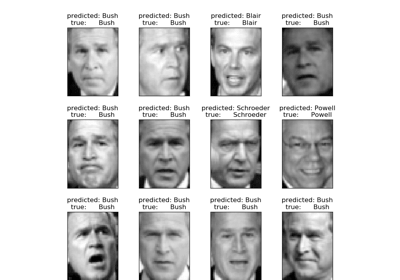Faces recognition example using eigenfaces and SVMs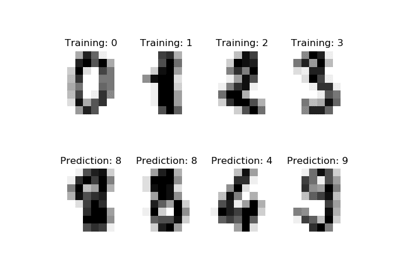Recognizing hand-written digitsColumn Transformer with Heterogeneous Data SourcesPipeline Anova SVMParameter estimation using grid search with cross-validation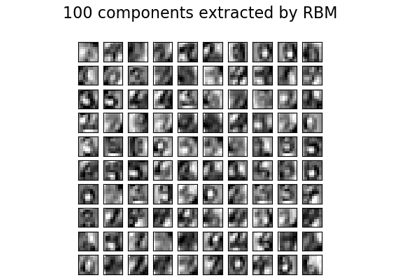Restricted Boltzmann Machine features for digit classification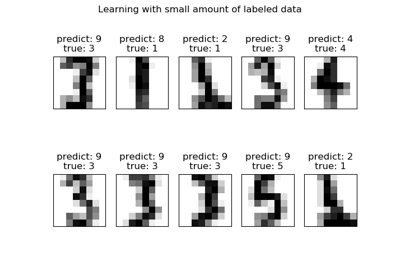Label Propagation digits: Demonstrating performance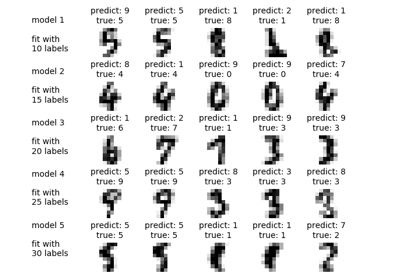Label Propagation digits active learning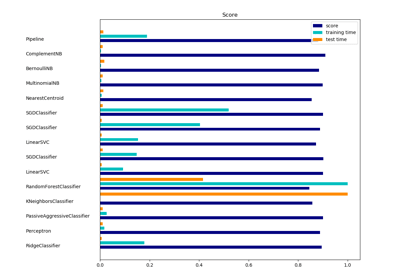Classification of text documents using sparse features